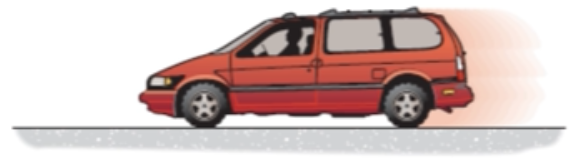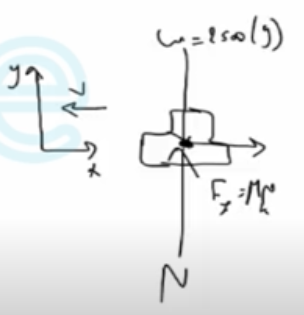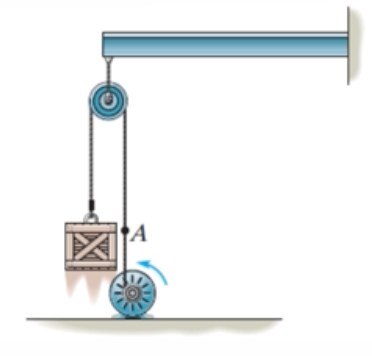Need Help?

Subscribe to Dynamic

###### \${selected_topic_name}
• Notes

$\begin{array}{l}{\text { 15-13. The 2.5-Mg van is traveling with a speed of } 100 \mathrm{km} / \mathrm{h}} \\ {\text { when the brakes are applied and all four wheels lock. If the }} \\ {\text { speed decreases to 40 } \mathrm{km} / \mathrm{h} \text { in 5 s, determine the coefficient }} \\ {\text { of kinetic friction between the tires and the road. }}\end{array}$$m=2.5 \mathrm{Mg}=2.5 \times 10^{6} \mathrm{g}$

$=2500 \mathrm{kg}$

$v_{1}=100 \mathrm{km} / \mathrm{h}$

$v_{2}=40 k{m} / h$

$t_2=5s \ , t_1=0$

$\mu_{k}=? ?$$v_{1}=100 * \frac{1000}{3600}=27.78m/ s, \ v_{2}=40 * \frac{1000}{3600}=11.11 m/ \mathrm{s}$

$(+ \uparrow y) ∴ m (v_{1})_{y}+\Sigma \int_{t_1}^{t_{2}}F_{y} d t= m (v_{2})_ y$

$2500(0)+[N(5-0)- 2500 (9.81)(5-1)]=2500 (0)$

$N=24525 \mathrm{N}$

$(\stackrel{+}{\leftarrow} x)∴ m\left(v_{1}\right)_{x}+\Sigma F_{x}\left(t_{2}-t_1\right)=m\left(v_{2}\right)_{x}$

$2500(27 . 78) +[-\mu _k(24525)(5-0)]=2500(11 . 11)$

$\mu_{k}=0.340$

$\begin{array}{l}{\text { 15-23. The motor pulls on the cable at } A \text { with a force }} \\ {F=\left(30+t^{2}\right) \text { lb, where } t \text { is in seconds. If the } 34-1 b \text { crate is }} \\ {\text { originally on the ground at } t=0 \text { , determine its speed in } t=4 \text { s. }} \\ {\text { Neglect the mass of the cable and pulleys. Hint: First find }} \\ {\text { the time needed to begin lifting the crate. }}\end{array}$$F=\left(30+t^{2}\right) lb$

$w=34lb$

$t^{\prime}=0$

$t_{2}=4s$$F=w \Rightarrow 30+t^{2}=34 \Rightarrow t^{2}=4 \Rightarrow t=2$

$(+ \uparrow) m v_{1}+\Sigma \int F d t=m v_{2}$

$0+\left[\int_{t_{1}}^{t_2} f d t-w\left(t_{2}-t_1\right)\right]=m v_{2}$

$0+\int_{2}^{4}\left(30+t^{2}\right) d t-34(4-2)=\frac{34}{32.2} v_{2}$

$\left[30 t+\frac{1}{3} t^{3}\right]_{2}^{4}-68=\frac{34}{32.2} \mathrm{V}_{2}$

$v_{2}=10.1 ft/s$